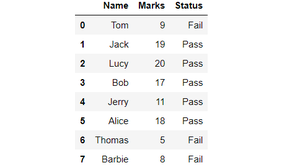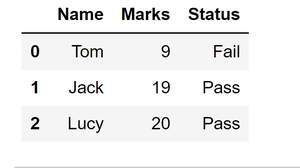# Get the first 3 rows of a given DataFrame

Let us first create a dataframe and then we will try to get first 3 rows of this dataframe using several methods.

Code: Creating a Dataframe.

## Python3

 `# import pandas library ` `import` `pandas as pd ` ` `  `# dictionary ` `record ``=` `{ ` `  ``"Name"``: [``"Tom"``, ``"Jack"``, ``"Lucy"``, ` `           ``"Bob"``, ``"Jerry"``, ``"Alice"``, ` `           ``"Thomas"``, ``"Barbie"``], ` `   `  `   ``"Marks"``: [``9``, ``19``, ``20``,  ` `             ``17``, ``11``, ``18``, ` `             ``5``, ``8``],  ` `   `  `  ``"Status"``: [``"Fail"``, ``"Pass"``, ``"Pass"``, ` `             ``"Pass"``,``"Pass"``, ``"Pass"``, ` `             ``"Fail"``, ``"Fail"``]} ` ` `  `# converting record into ` `# pandas dataframe ` `df ``=` `pd.DataFrame(record) ` ` `  `# printing whole dataframe ` `df`

Output:Output of above code: Dataframe created

## Getting first 3 Rows of the above Dataframe :

This method returns top n rows of the dataframe where n is an integer value and it specifies the number of rows to be displayed. The default value of n is 5 therefore, head function without arguments gives the first five rows of the dataframe as an output. So to get first three rows of the dataframe, we can assign the value of n as ‘3’.

Below is the code for getting first three rows of the dataframe using head() method:

## Python3

 `# import pandas library ` `import` `pandas as pd ` ` `  `# dictionary ` `record ``=` `{ ` `  ``"Name"``: [``"Tom"``, ``"Jack"``, ``"Lucy"``, ` `           ``"Bob"``, ``"Jerry"``, ``"Alice"``, ` `           ``"Thomas"``, ``"Barbie"``], ` `   `  `   ``"Marks"``: [``9``, ``19``, ``20``,  ` `             ``17``, ``11``, ``18``, ` `             ``5``, ``8``],  ` `   `  `  ``"Status"``: [``"Fail"``, ``"Pass"``, ``"Pass"``, ` `             ``"Pass"``,``"Pass"``, ``"Pass"``, ` `             ``"Fail"``, ``"Fail"``]} ` ` `  `# converting record into ` `# pandas dataframe ` `df ``=` `pd.DataFrame(record) ` ` `  `# select first 3 rows ` `# from the dataframe ` `df1 ``=` `df.head(``3``) ` ` `  `# show the dataframe ` `df1`

Output:Output of above code:- first three rows of the dataframe using head() function

Method 2: Using iloc[ ].

This can be used to slice a dataframe by using the starting index and ending index of the sliced dataframe that we want.

Syntax: dataframe.iloc[statrt_index, end_index+1]

So if we want first three rows, i.e. from index 0 to index 2, we can use the following code:

## Python3

 `# import pandas library ` `import` `pandas as pd ` ` `  `# dictionary ` `record ``=` `{ ` `  ``"Name"``: [``"Tom"``, ``"Jack"``, ``"Lucy"``, ` `           ``"Bob"``, ``"Jerry"``, ``"Alice"``, ` `           ``"Thomas"``, ``"Barbie"``], ` `   `  `   ``"Marks"``: [``9``, ``19``, ``20``,  ` `             ``17``, ``11``, ``18``, ` `             ``5``, ``8``],  ` `   `  `  ``"Status"``: [``"Fail"``, ``"Pass"``, ``"Pass"``, ` `             ``"Pass"``,``"Pass"``, ``"Pass"``, ` `             ``"Fail"``, ``"Fail"``]} ` ` `  `# converting record into ` `# pandas dataframe ` `df ``=` `pd.DataFrame(record) ` ` `  `# select first 3 rows ` `# from dataframe ` `df2 ``=` `df.iloc[``0``:``3``] ` ` `  `# show the dataframe ` `df2`Output of above code:- First three rows of the dataframe using iloc[] method

Method 3: Using index of the rows.

iloc[ ] method can also be used by directly stating the indices of the rows we want in the iloc method. Say to get row with indices m and n iloc[ ] can be used as:

Syntax: Dataframe.iloc [ [m,n] ]

Following is the code to get first three rows of the dataframe using this method:

## Python

 `# import pandas library ` `import` `pandas as pd ` ` `  `# dictionary ` `record ``=` `{ ` `  ``"Name"``: [``"Tom"``, ``"Jack"``, ``"Lucy"``, ` `           ``"Bob"``, ``"Jerry"``, ``"Alice"``, ` `           ``"Thomas"``, ``"Barbie"``], ` `   `  `   ``"Marks"``: [``9``, ``19``, ``20``,  ` `             ``17``, ``11``, ``18``, ` `             ``5``, ``8``],  ` `   `  `  ``"Status"``: [``"Fail"``, ``"Pass"``, ``"Pass"``, ` `             ``"Pass"``,``"Pass"``, ``"Pass"``, ` `             ``"Fail"``, ``"Fail"``]} ` ` `  `# converting record into ` `# pandas dataframe ` `df ``=` `pd.DataFrame(record) ` ` `  `# select first 3 rows  ` `# of the dataframe ` `df3 ``=` `df.iloc[[``0``, ``1``, ``2``]] ` ` `  `# show the dataframe ` `df3`

Output:Output of the above code:- First three rows of the dataframe using iloc and indices of the desired rows.

Whether you're preparing for your first job interview or aiming to upskill in this ever-evolving tech landscape, GeeksforGeeks Courses are your key to success. We provide top-quality content at affordable prices, all geared towards accelerating your growth in a time-bound manner. Join the millions we've already empowered, and we're here to do the same for you. Don't miss out - check it out now!

Previous
Next